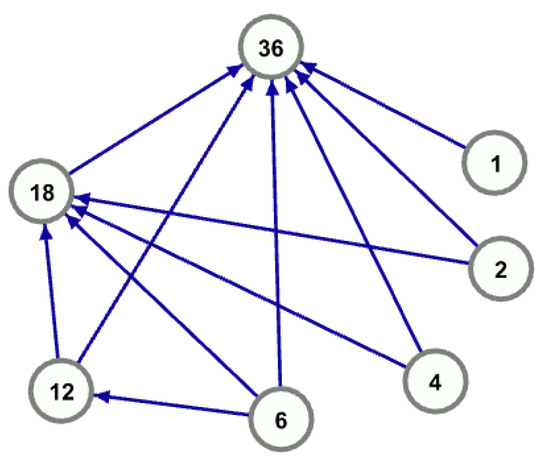Next Article in Journal
A Novel Approach for Web Service Recommendation Based on Advanced Trust Relationships
Open AccessArticle

# Idempotent Factorizations of Square-Free Integers

byBarry FaginDepartment of Computer Science, US Air Force Academy, Colorado Springs, CO 80840, USA
Information 2019, 10(7), 232; https://doi.org/10.3390/info10070232
Received: 20 June 2019 / Accepted: 3 July 2019 / Published: 6 July 2019
We explore the class of positive integers n that admit idempotent factorizations $n = p ¯ q ¯$ such that $λ ( n ) ∣ ( p ¯ − 1 ) ( q ¯ − 1 )$ , where $λ$ is the Carmichael lambda function. Idempotent factorizations with $p ¯$ and $q ¯$ prime have received the most attention due to their cryptographic advantages, but there are an infinite number of n with idempotent factorizations containing composite $p ¯$ and/or $q ¯$ . Idempotent factorizations are exactly those $p ¯$ and $q ¯$ that generate correctly functioning keys in the Rivest–Shamir–Adleman (RSA) 2-prime protocol with n as the modulus. While the resulting $p ¯$ and $q ¯$ have no cryptographic utility and therefore should never be employed in that capacity, idempotent factorizations warrant study in their own right as they live at the intersection of multiple hard problems in computer science and number theory. We present some analytical results here. We also demonstrate the existence of maximally idempotent integers, those n for which all bipartite factorizations are idempotent. We show how to construct them, and present preliminary results on their distribution. View Full-Text
Show FiguresFigure 1

MDPI and ACS Style

Fagin, B. Idempotent Factorizations of Square-Free Integers. Information 2019, 10, 232.